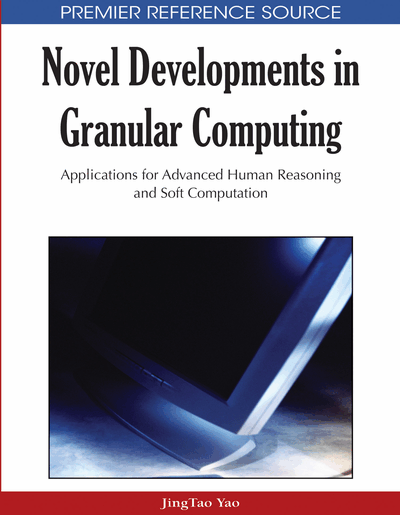# Rough Entropy Clustering Algorithm in Image Segmentation

Dariusz Malyszko (Bialystok University of Technology, Poland) and Jaroslaw Stepaniuk (Bialystok University of Technology, Poland)
DOI: 10.4018/978-1-60566-324-1.ch012
Available
\$33.75
List Price: \$37.50
10% Discount:-\$3.75
TOTAL SAVINGS: \$3.75

## Abstract

Clustering understood as a data grouping technique represents fundamental procedures in image processing. The present chapter’s concerns are combining the concept of rough sets and entropy measures in the area of image segmentation. In this context, comprehensive investigations into rough set entropy based clustering image segmentation techniques have been performed. Segmentation presents low-level image transformation routines concerned with image partitioning into distinct disjoint and homogenous regions. In the area of segmentation routines, threshold based algorithms and clustering algorithms most often are applied in practical solutions when there is a pressing need for simplicity and robustness. Rough entropy threshold based segmentation algorithms simultaneously combine optimal threshold determination with rough region approximations and region entropy measures. In the present chapter, new algorithmic schemes RECA in the area of rough entropy based partitioning routines have been proposed. Rough entropy clustering incorporates the notion of rough entropy into clustering models, taking advantage of dealing with some degree of uncertainty in analyzed data. RECA algorithmic schemes performed usually equally robust compared to standard k-means algorithms. At the same time, in many runs they yielded slightly better performances making possible future implementation in clustering applications.
Chapter Preview
Top

## 1. Introduction

During last decades, constantly growing research attention has been focused on data clustering as fundamental technique in data analysis. Clustering or data grouping describes important method of unsupervised classification that arranges pattern data, most often vectors in multidimensional space, in the clusters or groups. Patterns or vectors in the same cluster are similar according to predefined criteria, in contrast to distinct patterns from different clusters (Jain, 1989) (Xu, Wunsch, 2005). Clustering algorithms are widely recognized as robust, high quality techniques of data analysis. Possible areas of application of clustering algorithms include data mining, statistical data analysis, compression, vector quantization and pattern recognition(Jain, 1989). Image analysis is the area where grouping data into meaningful regions (referred to as image segmentation) presents the first step into more detailed problem specific routines and procedures in computer vision and image understanding.

There is a growing need for effective segmentation routines capable of handling emerging different types of novel imagery suitable particularly for their distinct characteristics and taking into account their area of application. High quality of image segmentation requires incorporating reasonably as much information of an image as possible to retrieve. This kind of combining diverse information in a segmentation understood as a means of improving algorithm performance has been widely recognized and acknowledged in the literature of the subject.

In practical applications, most often image regions do not depict well-defined homogeneous characteristics, so it seems naturally appropriate to use techniques that additionally incorporate the ambiguity in information for performing the thresholding operation. In recent years (Pawlak, Skowron, 2007) (Pawlak, 1991) the theory of rough sets has gained considerable importance with numerous applications in diverse areas of research, especially in data mining, knowledge discovery, artificial intelligence and information systems analysis. Combination of thresholding methods with rough set theory has been introduced in (Pal, Shankar, Mitra, 2005). The authors minimize the roughness value in order to perform image thresholding by optimizing an entropy measure, which they refer to as the ”rough entropy of image”. Rough entropy measure calculation of the cluster centers is based on lower and upper approximation generated by assignments data objects to the cluster centers. Roughness of the cluster center is calculated from lower and upper approximations of each cluster center. In the next step, rough entropy is calculated as the sum of all entropies of cluster center roughness values. Higher roughness measure value describes the cluster model with more uncertainty at the border. Uncertainty of the class border should be possibly high as opposed to the class lower approximation. For each selected cluster centers, rough entropy measure determines quality measure or fitness value of this cluster centers. In this way, combining clustering schemes with uncertainty and entropy handling by means of rough entropy in the area of image segmentation should take best advantages of all described techniques.

### 1.1. Motivation

The present study proposes and thoroughly investigates new granular rough entropy method in the area of image clustering that extends and supplements algorithm proposed in (Pal, Shankar, Mitra, 2005), one-dimensional 1D GMRET thresholding algorithm proposed in (Malyszko, Stepaniuk, n.d.) and two-dimensional 2D GMRET thresholding algorithm in (Malyszko, Stepaniuk, 2008). Maximization of rough entropy of the image results in maximization of roughness in multiple clustering areas - providing the optimal threshold value of partitioning. The present study contribution consists in extension of rough entropy based threshold calculation schemes, which from definition are confined to low dimensional data into clustering schemes capable of handling high dimensional data. Clustering routines are more sophisticated and robust techniques that generally better describe underlying data structure compared to threshold based segmentation procedures.

## Complete Chapter List

Search this Book:
Reset